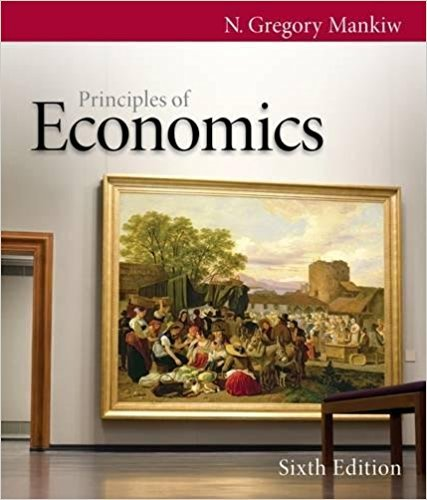×
Get Full Access to Principles Of Economics - 6 Edition - Chapter 4 - Problem Problems and applications 4.14
Get Full Access to Principles Of Economics - 6 Edition - Chapter 4 - Problem Problems and applications 4.14

×

Textbooks / Business / Principles of Economics 6 / Chapter 4 / Problem Problems and Applications 4.14

# Market research has revealed the following information about the market for chocolateISBN: 9780538453059 472

## Solution for problem Problems and Applications 4.14 Chapter 4

Principles of Economics | 6th Edition

• Textbook Solutions
• 2901 Step-by-step solutions solved by professors and subject experts
• Get 24/7 help from StudySoup virtual teaching assistantsPrinciples of Economics | 6th Edition

4 5 1 285 Reviews
18
0
Problem Problems and Applications 4.14

Market research has revealed the following information about the market for chocolate bars: The demand schedule can be represented by the equation QD = 1,600 300P, where QD is the quantity demanded and P is the price. The supply schedule can be represented by the equation QS = 1,400 + 700P, where QS is the quantity supplied. Calculate the equilibrium price and quantity in the market for chocolate bars.

Step-by-Step Solution:

Step 1 of 2

The quantity demanded and quantity supplied of a commodity depends upon the price of the commodity. Where demand establishes a negative relationship with price and supply establishes a positive relationship with price.

Step 2 of 2

##### ISBN: 9780538453059

Since the solution to Problems and Applications 4.14 from 4 chapter was answered, more than 230 students have viewed the full step-by-step answer. Principles of Economics was written by and is associated to the ISBN: 9780538453059. This full solution covers the following key subjects: . This expansive textbook survival guide covers 36 chapters, and 670 solutions. The answer to “Market research has revealed the following information about the market for chocolate bars: The demand schedule can be represented by the equation QD = 1,600 300P, where QD is the quantity demanded and P is the price. The supply schedule can be represented by the equation QS = 1,400 + 700P, where QS is the quantity supplied. Calculate the equilibrium price and quantity in the market for chocolate bars.” is broken down into a number of easy to follow steps, and 69 words. The full step-by-step solution to problem: Problems and Applications 4.14 from chapter: 4 was answered by , our top Business solution expert on 03/16/18, 04:26PM. This textbook survival guide was created for the textbook: Principles of Economics, edition: 6.

Unlock Textbook Solution